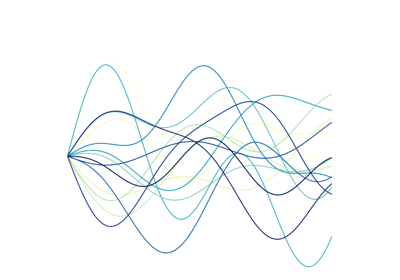# tslearn.generators.random_walk_blobs¶

tslearn.generators.random_walk_blobs(n_ts_per_blob=100, sz=256, d=1, n_blobs=2, noise_level=1.0, random_state=None)[source]

Blob-based random walk time series generator.

Generate n_ts_per_blobs * n_blobs time series of size sz and dimensionality d. Generated time series follow the model:

$ts[t] = ts[t - 1] + a$

where $$a$$ is drawn from a normal distribution of mean mu and standard deviation std.

Each blob contains time series derived from a same seed time series with added white noise.

Parameters: n_ts_per_blob : int (default: 100) Number of time series in each blob sz : int (default: 256) Length of time series (number of time instants) d : int (default: 1) Dimensionality of time series n_blobs : int (default: 2) Number of blobs noise_level : float (default: 1.) Standard deviation of white noise added to time series in each blob random_state : integer or numpy.RandomState or None (default: None) Generator used to draw the time series. If an integer is given, it fixes the seed. Defaults to the global numpy random number generator. numpy.ndarray A dataset of random walk time series numpy.ndarray Labels associated to random walk time series (blob id)

Examples

>>> X, y = random_walk_blobs(n_ts_per_blob=100, sz=256, d=5, n_blobs=3)
>>> X.shape
(300, 256, 5)
>>> y.shape
(300,)


## Examples using tslearn.generators.random_walk_blobs¶Nearest neighbors